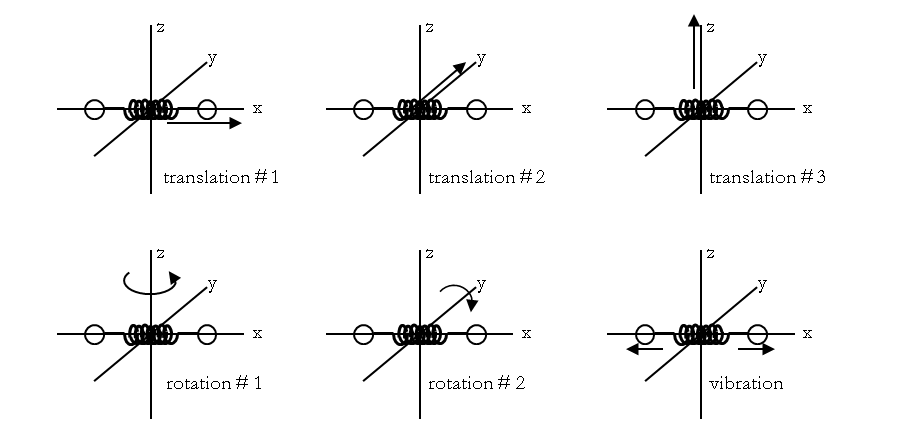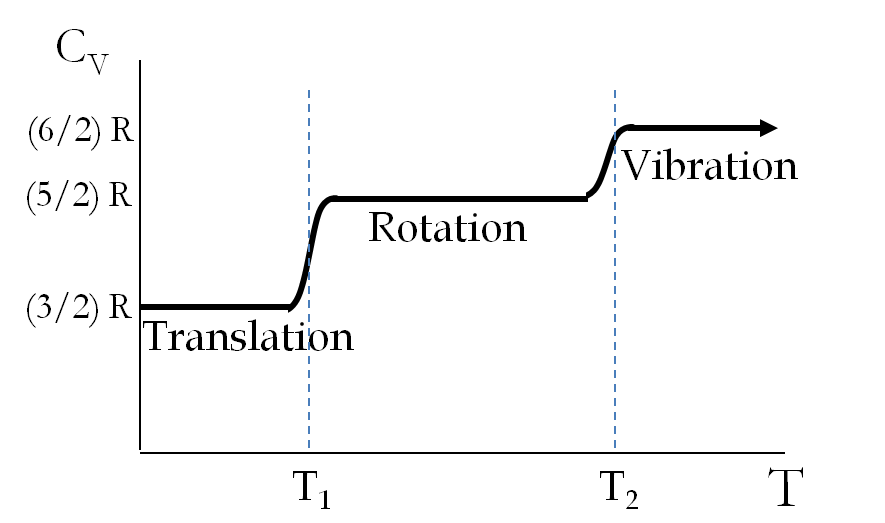## Section27.4Internal Energy of Ideal Gases

The kinetic theory can be used to calculate the internal energy of an ideal gas. The internal energy of a collection of gas molecules will be equal to the sum of the average kinetic and potential energies of all molecules. Since the molecules of a monatomic ideal gas do not interact with each other, they do not possess any potential energy. The molecules of monatomic ideal gas have only kinetic energy.

The average kinetic energy of one molecule is

\begin{equation*} \text{KE} = \frac{1}{2}m v_\text{rms}^2. \end{equation*}

The quantity $v_\text{rms}$ is the root-mean square speed, which is given by the following expression based on Maxwell velocity distribution.

\begin{equation*} v_{rms}^2 = \frac{3k_BT}{m}. \end{equation*}

Therefore, kinetic energy of one molecule is

\begin{equation*} \text{KE} = \frac{3}{2}k_BT. \end{equation*}

If the monatomic ideal gas has $N$ molecules, the internal energy $U$ of the gas will be

\begin{equation*} U = \frac{3}{2}Nk_BT \end{equation*}

This shows that internal energy of an ideal gas only depends on the amount of the gas and the temperature. We can write this equation in term of number of moles $n\text{,}$ which is $N/N_A\text{,}$ where $N_A$ is Avogadro's number. When writing the formula in moles, the product $k_BN_A$ is normally replaced with the gas constant $R \text{.}$

\begin{equation} U = 3\times \frac{1}{2}nRT\tag{27.4.1} \end{equation}

The factor 3 in these equations comes from three translational degrees of motion for each molecule. Each translational degree of freedom contributes $\frac{1}{2} k_BT$ per molecule equally. This result is known as the quipartition of energy.

### Subsection27.4.1Internal Energy and Degrees of Freedom

We saw that the internal energy of monatomic ideal gas comes from the sum of contributions from three degrees of translational motion for each molecule. {\bf Degrees of freedom}\index{Degrees of freedom} of a particle refer to the number of coordinates required to specify the position of the particle. A point-like molecule, such as monatomic ideal gas molecule, has only the translational degrees of motion in three-dimensional space. This gives them three degrees of freedom.

If molecules of the system contain more than one atom per molecule, there will be additional degrees of motion, such as rotation and vibration, which will add to the total kinetic energy of each molecule. For molecules with $A$ atoms, we need $3\times A$ coordinates, which could be $(x,y,z)$ for each atom. Therefore, a molecule with $A$ atoms will have a total of $3A$ degrees of freedom. These coordinates can be combined to yield three coordinates of the center of mass, three rotation angles if the molecule is not linear otherwise two rotation angles, and the rest correspond to vibrations of the molecule.

The molecule can be moved about in any of the three translational motion of the center of mass with any arbitrarily small energy, but excitation of rotation and vibration motions require a minimum energy. If temperature is high enough so that $k_BT$ is more than what is required to excite a particular mode of motion, then that mode will also contribute to the internal energy. Each degree of freedom that participates in the motion contributes $\frac{1}{2}k_BT$ to the internal energy.

For instance, the molecules of a diatomic gas have two atoms in each molecule. Of the six degrees of freedom of a diatomic molecule, one is vibrational. Six modes of a diatomic molecule are shown in Figure 27.4.1.Figure 27.4.1. Six modes of motion in a diatomic molecule. The translational, rotational and vibrational modes are turned on at different temperatures.

The vibration of molecules is usually modeled by placing a fictitious spring between the atoms which represent chemical bonds between them. Therefore, when a vibrational degree of freedom is excited, we also must include a potential energy of each molecule due to the “spring-like” binding force between the atoms of a diatomic molecule. According to the virial theorem, the average potential and the average kinetic energies in a spring oscillation are equal to each other. Therefore, the average potential energy for a vibrating mode will be:

\begin{equation*} \text{Average energy per excited vibration mode } = \frac{1}{2}k_B T. \end{equation*}

Thus, the internal energy of an ideal gas comes from the kinetic energy of the translation and rotation modes and the potential energy of chemical bonds if vibration is an available mode at the temperature under consideration. Let $f$ be the total number of translation, rotation, and vibration modes in the molecule that are excited at the temperature of the gas. Then, the internal energy of $N$ molecules of the gas at absolute temperature $T$ will be

\begin{equation*} U = f\times\frac{1}{2}k_B T. \end{equation*}

At very low temperature such as 50 K, normally only translational modes are excited and then $f = 3\text{.}$ At very high temperature such as 1000 K all six modes, three translational, two rotational and one vibrational, are excited therefore $f =6\text{.}$ Thus, a diatomic ideal gas has $U=6/2 k_BT$ per molecule at high temperatures while $U=3/2 k_B T$ at low temperatures.

Recall that specific heat is the rate at which internal energy changes with temperature. In particular specific heat at constant volume gives an indication of the number of modes that are turned on at any temperature. In Figure 27.4.2 shows a schematic plot of the specific heat at constant volume versus temperature. The plot shows the contributions of modes of motion that are turned on as temperature is raised. Once, the maximum number of modes, viz. $3\times A$ for an $A$-atom molecule, are turned on, the specific heat would plateau out.Figure 27.4.2. Specific heat of a diatomic ideal gas as a function of temperature showing exciting of rotation and then vibrational modes as temperature is raised.